# 一种基于ALD和AEP分布的贝叶斯分位数回归A Bayesian Quantile Regression Based on ALD and AEP Distribution

DOI: 10.12677/AAM.2020.97125, PDF, HTML, XML, 下载: 151  浏览: 373

Abstract: In this paper, the asymmetric exponential power distribution (AEP) and asymmetric Laplace distribution (ALD) are used to make assumptions about the error terms of the quantile regression model, and the parameters of Bayesian quantile regression are estimated for this. Aiming at the complexity of the posterior density of parameters, the Gibbs sampling algorithm is used to sample the posterior parameters of the ALD distribution and AEP distribution. According to the numerical simulation results, the error following the AEP distribution is assumed to be more adaptable to the data than the ALD distribution.

1. 引言

1978年Koenker首次提出“分位数回归”概念 ，用于解释因变量与自变量之间的关系。1999年Koenker和Machado研究了非对称拉普拉斯分布和分位数回归之间的关系 。2001年Keming Yu  介绍了一种贝叶斯分位数回归思想，采用基于非对称拉普拉斯分布对参数进行估计，并证实无论数据的原始分布如何，使用非对称拉普拉斯分布对贝叶斯分位数回归建模是一种非常自然且有效的方法。自此，贝叶斯估计方法在分位数回归中有越来越多的应用。

2. 非对称拉普拉斯分布与非对称指数幂分布

$f\left(y;\mu ,\sigma ,p\right)=\frac{p\left(1-p\right)}{\sigma }\mathrm{exp}\left\{-\frac{y-\mu }{\sigma }\left[p-I\left(y\le \mu \right)\right]\right\},y\in \left(-\infty ,+\infty \right)$ (1)

${f}_{\text{AEP}}\left(y|\mu ,\sigma ,\alpha ,{p}_{1}{p}_{2}\right)=\left\{\begin{array}{l}\frac{1}{\sigma }\mathrm{exp}\left(-{|\frac{y-\mu }{\alpha \sigma /\Gamma \left(1+{p}_{1}\right)}|}^{{p}_{1}}\right),y\le \mu \\ \frac{1}{\sigma }\mathrm{exp}\left(-{|\frac{y-\mu }{\left(1-\alpha \right)\sigma /\Gamma \left(1+{p}_{2}\right)}|}^{{p}_{2}}\right),y>\mu \end{array}$ (2)

3. 贝叶斯分位数回归

3.1. 设线性回归模型

${y}_{i}={\beta }_{1}{x}_{i1}+{\beta }_{2}{x}_{i2}+\cdots +{\beta }_{k}{x}_{ik}+{\epsilon }_{i},\text{\hspace{0.17em}}\text{\hspace{0.17em}}i=1,\cdots ,n;\text{\hspace{0.17em}}k=1,\cdots ,n$ (3)

3.2. 非对称拉普拉斯分布似然函数(ALD)

$L\left({y}_{i}|{x}_{i}^{\text{T}}\beta ,\sigma ,p\right)=\frac{{p}^{n}{\left(1-p\right)}^{n}}{{\sigma }^{n}}\mathrm{exp}\left\{-\frac{1}{\sigma }\underset{i}{\sum }{\rho }_{p}\left({y}_{i}-{x}_{i}^{\text{T}}\beta \right)\right\}$ (4)

3.3. 非对称指数幂分布似然函数(AEPD)

Naranjo在2012  和2015  年对SEP分布和AEP分布进行相关的研究，提出了关于非对称指数幂相关的理论和方法。

$Y|\left[{U}_{1}={u}_{1}\right]~U\left(\mu -\frac{\alpha \sigma }{\Gamma \left(1+\frac{1}{{p}_{1}}\right)}{u}_{1}^{\frac{1}{{p}_{1}}},\mu \right)$${U}_{1}~Ga\left(1+\frac{1}{{p}_{1}},1\right)$，且概率为 $\alpha$，和 $Y|\left[{U}_{2}={u}_{2}\right]~U\left(\mu ,\mu +\frac{\left(1-\alpha \right)\sigma }{\Gamma \left(1+\frac{1}{{p}_{2}}\right)}{u}_{2}^{\frac{1}{{p}_{2}}}\right)$${U}_{2}~Ga\left(1+\frac{1}{{p}_{2}},1\right)$，且概率为 $\left(1-\alpha \right)$，则 $Y~\text{AEP}\left(\mu ,\sigma ,\alpha ,{p}_{1},{p}_{2}\right)$

$\begin{array}{l}L\left(y,x,{u}_{1},{u}_{2}|\beta ,\sigma ,\alpha ,{p}_{1},{p}_{2}\right)\\ =\underset{i=1}{\overset{n}{\sum }}\frac{1}{\sigma }\left\{\mathrm{exp}\left(-{u}_{1}\right)I\left[{x}_{i}^{\text{T}}\beta -\frac{\alpha \sigma }{\Gamma \left(1+\frac{1}{{p}_{1}}\right)}{u}_{1}^{\frac{1}{{p}_{1}}}<{y}_{i}<{x}_{i}^{\text{T}}\beta \right]\\ \text{\hspace{0.17em}}\text{\hspace{0.17em}}\text{ }\text{ }+\mathrm{exp}\left(-{u}_{2}\right)I\left[{x}_{i}^{\text{T}}\beta <{y}_{i}<{x}_{i}^{\text{T}}\beta +\frac{\left(1-\alpha \right)\sigma }{\Gamma \left(1+\frac{1}{{p}_{2}}\right)}{u}_{2}^{\frac{1}{{p}_{2}}}\right]\right\}\end{array}$ (5)

$\begin{array}{l}P\left({u}_{1},{u}_{2},\beta ,\sigma ,\alpha ,{p}_{1},{p}_{2}|y,x\right)\\ \propto f\left(\beta \right)f\left(\sigma \right)f\left(\alpha \right)f\left({p}_{1}\right)f\left({p}_{2}\right){\sigma }^{-n}\\ \text{\hspace{0.17em}}\text{\hspace{0.17em}}\text{\hspace{0.17em}}×\underset{i=1}{\overset{n}{\sum }}\left(\mathrm{exp}\left(-{u}_{1}\right)\underset{i=1}{\overset{n}{\sum }}\frac{1}{\sigma }\left\{\mathrm{exp}\left(-{u}_{1}\right)I\left[{x}_{i}^{\text{T}}\beta -\frac{\alpha \sigma }{\Gamma \left(1+\frac{1}{{p}_{1}}\right)}{u}_{1}^{\frac{1}{{p}_{1}}}<{y}_{i}<{x}_{i}^{\text{T}}\beta \right]\\ \text{\hspace{0.17em}}\text{\hspace{0.17em}}\text{\hspace{0.17em}}+\mathrm{exp}\left(-{u}_{2}\right)I\left[{x}_{i}^{\text{T}}\beta <{y}_{i}<{x}_{i}^{\text{T}}\beta +\frac{\left(1-\alpha \right)\sigma }{\Gamma \left(1+\frac{1}{{p}_{2}}\right)}{u}_{2}^{\frac{1}{{p}_{2}}}\right]\right\}\right)\end{array}$ (6)

$\left\{\begin{array}{l}{u}_{1},{u}_{2}~Exp\left(1\right)\\ \sigma ~IG\left({a}_{\sigma },{b}_{\sigma }\right)\\ \alpha ~Beta\left({a}_{\alpha },{b}_{\alpha }\right)\\ {p}_{1},{p}_{2}~N\left({a}_{{p}_{i}},{b}_{{p}_{i}}\right)\\ \beta ~{N}_{k}\left(b,B\right)\end{array}$ (7)

1) ${u}_{1},{u}_{2}$

$\begin{array}{l}P\left({u}_{1i}|y,x,\beta ,\sigma ,\alpha ,{p}_{1},{p}_{2}\right)\\ \propto f\left({u}_{1i}\right)I\left[{u}_{1i}>{\left(\frac{\left({x}_{i}^{\text{T}}\beta -{y}_{i}\right)\text{Γ}\left(1+\frac{1}{{p}_{1}}\right)}{\alpha \sigma }\right)}^{{p}_{1}}\right]\left({y}_{i}<{x}_{i}^{\text{T}}\beta \right)\\ \propto Exp\left(1\right)I\left[{u}_{1i}>{\left(\frac{\left({x}_{i}^{\text{T}}\beta -{y}_{i}\right)\text{Γ}\left(1+\frac{1}{{p}_{1}}\right)}{\alpha \sigma }\right)}^{{p}_{1}}\right]\left({y}_{i}<{x}_{i}^{\text{T}}\beta \right)\end{array}$ (8)

$\begin{array}{l}P\left({u}_{2i}|y,x,\beta ,\sigma ,\alpha ,{p}_{1},{p}_{2}\right)\\ \propto f\left({u}_{2i}\right)I\left[{u}_{2i}>{\left(\frac{\left({y}_{i}-{x}_{i}^{\text{T}}\beta \right)\Gamma \left(1+\frac{1}{{p}_{2}}\right)}{\left(1-\alpha \right)\sigma }\right)}^{{p}_{2}}\right]\left({y}_{i}>{x}_{i}^{\text{T}}\beta \right)\\ \propto Exp\left(1\right)I\left[{u}_{2i}>{\left(\frac{\left({y}_{i}-{x}_{i}^{\text{T}}\beta \right)\Gamma \left(1+\frac{1}{{p}_{2}}\right)}{\left(1-\alpha \right)\sigma }\right)}^{{p}_{2}}\right]\left({y}_{i}>{x}_{i}^{\text{T}}\beta \right)\end{array}$ (9)

2) $\sigma$

$\begin{array}{l}P\left(\sigma |y,x,{u}_{1},{u}_{2},\alpha ,\beta ,{p}_{1},{p}_{2}\right)\\ \propto f\left(\sigma \right)\frac{1}{{\sigma }^{n}}I\left[\sigma >\underset{_}{\sigma }\right]\\ \propto IG\left(n-1+{a}_{\sigma },{b}_{\sigma }\right)I\left[\sigma >\underset{_}{\sigma }\right]\end{array}$ (10)

${u}_{1i}>0$ 时， ${x}_{i}^{\text{T}}\beta -\frac{\alpha \sigma }{\Gamma \left(1+\frac{1}{{p}_{1}}\right)}{u}_{1i}^{\frac{1}{{p}_{1}}}<{y}_{i}$，化简为：

$\sigma >\frac{\left({x}_{i}^{\text{T}}\beta -{y}_{i}\right)\Gamma \left(1+\frac{1}{{p}_{1}}\right)}{\alpha {u}_{1i}^{1/{p}_{1}}}$

${u}_{2i}>0$ 时， ${y}_{i}<{x}_{i}^{\text{T}}\beta +\frac{\left(1-\alpha \right)\sigma }{\Gamma \left(1+\frac{1}{{p}_{2}}\right)}{u}_{2}^{\frac{1}{{p}_{2}}}$，化简为：

$\sigma >\frac{\left({y}_{i}-{x}_{i}^{\text{T}}\beta \right)\Gamma \left(1+\frac{1}{{p}_{2}}\right)}{\left(1-\alpha \right){u}_{2i}^{1/{p}_{2}}}$

3) $\alpha$

$P\left(\alpha |y,x,{u}_{1},{u}_{2},\sigma ,\beta ,{p}_{1},{p}_{2}\right)\propto f\left(\alpha \right)I\left[\underset{_}{\alpha }<\alpha <\stackrel{¯}{\alpha }\right]\propto Beta\left({a}_{\alpha },{b}_{\alpha }\right)I\left[\underset{_}{\alpha }<\alpha <\stackrel{¯}{\alpha }\right]$ (11)

${u}_{1i}>0,{u}_{2i}>0$ 时，有 ${x}_{i}^{\text{T}}\beta -\frac{\alpha \sigma }{\text{Γ}\left(1+\frac{1}{{p}_{1}}\right)}{u}_{1i}^{\frac{1}{{p}_{1}}}<{y}_{i}<{x}_{i}^{\text{T}}\beta +\frac{\left(1-\alpha \right)\sigma }{\text{Γ}\left(1+\frac{1}{{p}_{2}}\right)}{u}_{2}^{\frac{1}{{p}_{2}}}$

$\underset{_}{\alpha }=\mathrm{max}\left\{0,\frac{\left({x}_{i}^{\text{T}}\beta -{y}_{i}\right)\text{Γ}\left(1+\frac{1}{{p}_{1}}\right)}{\sigma {u}_{1i}^{\frac{1}{{p}_{1}}}}\right\}$

$\stackrel{¯}{\alpha }=\mathrm{min}\left\{0,1-\frac{\left({y}_{i}-{x}_{i}^{\text{T}}\beta \right)\text{Γ}\left(1+\frac{1}{{p}_{2}}\right)}{\sigma {u}_{2i}^{1/{p}_{2}}}\right\}$

4) ${p}_{1},{p}_{2}$

$\begin{array}{l}P\left({p}_{1}|y,x,{u}_{1},{u}_{2},\alpha ,\sigma ,\beta ,{p}_{2}\right)\\ \propto f\left({p}_{1}\right)I\left[\frac{{x}_{i}^{\text{T}}\beta -{y}_{i}}{\alpha \sigma }<\frac{{u}_{1i}^{1/{p}_{1}}}{\text{Γ}\left(1+\frac{1}{{p}_{1}}\right)}\right]\left({u}_{1i}>0\right)\\ \propto N\left({a}_{{p}_{1}},{b}_{{p}_{1}}\right)I\left[\frac{{x}_{i}^{\text{T}}\beta -{y}_{i}}{\alpha \sigma }<\frac{{u}_{1i}^{1/{p}_{1}}}{\text{Γ}\left(1+\frac{1}{{p}_{1}}\right)}\right]\left({u}_{1i}>0\right)\end{array}$ (12)

$\begin{array}{l}P\left({p}_{2}|y,x,{u}_{1},{u}_{2},\alpha ,\sigma ,\beta ,{p}_{1}\right)\\ \propto f\left({p}_{2}\right)I\left[\frac{{y}_{i}-{x}_{i}^{\text{T}}\beta }{\left(1-\alpha \right)\sigma }<\frac{{u}_{2i}^{1/{p}_{2}}}{\text{Γ}\left(1+\frac{1}{{p}_{2}}\right)}\right]\left({u}_{2i}>0\right)\\ \propto N\left({a}_{{p}_{2}},{b}_{{p}_{2}}\right)I\left[\frac{{y}_{i}-{x}_{i}^{\text{T}}\beta }{\left(1-\alpha \right)\sigma }<\frac{{u}_{2i}^{1/{p}_{2}}}{\text{Γ}\left(1+\frac{1}{{p}_{2}}\right)}\right]\left({u}_{2i}>0\right)\end{array}$ (13)

5) $\beta$

${\beta }_{-j}^{\text{T}}=\left({\beta }_{1},\cdots ,{\beta }_{j-1},{\beta }_{j+1},\cdots ,{\beta }_{k}\right)$ 为不含第j个参数的向量， $\beta$ 的先验分布服从多元正态分布，根据贝叶斯定理和多元正态分布性质可得：

$\begin{array}{l}P\left({\beta }_{j}|y,x,{u}_{1},{u}_{2},{\beta }_{-j},\alpha ,\sigma ,\beta ,{p}_{1},{p}_{2}\right)\\ \propto f\left({\beta }_{j}\right)I\left[{\beta }_{j}\in \left({\underset{_}{\beta }}_{j},{\stackrel{¯}{\beta }}_{j}\right)\right]\propto N\left({b}_{j}^{*},{B}_{j}^{*}\right)I\left[{\beta }_{j}\in \left({\underset{_}{\beta }}_{j},{\stackrel{¯}{\beta }}_{j}\right)\right]\end{array}$ (14)

${x}_{i}^{\text{T}}\beta -\frac{\alpha \sigma }{\text{Γ}\left(1+\frac{1}{{p}_{1}}\right)}{u}_{1}^{\frac{1}{{p}_{1}}}<{y}_{i}<{x}_{i}^{\text{T}}\beta +\frac{\left(1-\alpha \right)\sigma }{\text{Γ}\left(1+\frac{1}{{p}_{2}}\right)}{u}_{2}^{\frac{1}{{p}_{2}}}$

${x}_{ij}>0$ 时，化简为：

$\frac{{y}_{i}-{x}_{i\left(-j\right)}^{\text{T}}{\beta }_{\left(-j\right)}}{{x}_{ij}}-\frac{\left(1-\alpha \right)\sigma {u}_{2i}^{1/{p}_{2}}}{{x}_{ij}\text{Γ}\left(1+1/{p}_{2}\right)}<{\beta }_{j}<\frac{{y}_{i}-{x}_{i\left(-j\right)}^{\text{T}}{\beta }_{\left(-j\right)}}{{x}_{ij}}+\frac{\alpha \sigma {u}_{1i}^{1/{p}_{1}}}{{x}_{ij}\text{Γ}\left(1+1/{p}_{1}\right)}$

${x}_{ij}<0$ 时，化简为：

$\frac{{y}_{i}-{x}_{i\left(-j\right)}^{\text{T}}{\beta }_{\left(-j\right)}}{{x}_{ij}}+\frac{\alpha \sigma {u}_{1i}^{1/{p}_{1}}}{{x}_{ij}\text{Γ}\left(1+1/{p}_{1}\right)}<{\beta }_{j}<\frac{{y}_{i}-{x}_{i\left(-j\right)}^{\text{T}}{\beta }_{\left(-j\right)}}{{x}_{ij}}-\frac{\left(1-\alpha \right)\sigma {u}_{2i}^{1/{p}_{2}}}{{x}_{ij}\text{Γ}\left(1+1/{p}_{2}\right)}$

${\underset{_}{\beta }}_{j}=\left\{\frac{{y}_{i}-{x}_{i\left(-j\right)}^{\text{T}}{\beta }_{\left(-j\right)}}{{x}_{ij}}-\frac{\left(1-\alpha \right)\sigma {u}_{2i}^{\frac{1}{{p}_{2}}}}{{x}_{ij}\text{Γ}\left(1+\frac{1}{{p}_{2}}\right)},\frac{{y}_{i}-{x}_{i\left(-j\right)}^{\text{T}}{\beta }_{\left(-j\right)}}{{x}_{ij}}+\frac{\alpha \sigma {u}_{1i}^{1/{p}_{1}}}{{x}_{ij}\text{Γ}\left(1+1/{p}_{1}\right)}\right\}$

${\stackrel{¯}{\beta }}_{j}=\left\{\frac{{y}_{i}-{x}_{i\left(-j\right)}^{\text{T}}{\beta }_{\left(-j\right)}}{{x}_{ij}}+\frac{\alpha \sigma {u}_{1i}^{\frac{1}{{p}_{1}}}}{{x}_{ij}\text{Γ}\left(1+\frac{1}{{p}_{1}}\right)},\frac{{y}_{i}-{x}_{i\left(-j\right)}^{\text{T}}{\beta }_{\left(-j\right)}}{{x}_{ij}}-\frac{\left(1-\alpha \right)\sigma {u}_{2i}^{1/{p}_{2}}}{{x}_{ij}\text{Γ}\left(1+1/{p}_{2}\right)}\right\}$

4. 数据模拟分析

$\left\{\begin{array}{l}{y}_{i}={\beta }_{1}{x}_{1}+{\beta }_{2}{x}_{2}+{\epsilon }_{i},\text{\hspace{0.17em}}i=1,2,\cdots ,n\\ {x}_{i}~unif\left(0,100\right)\\ {\beta }_{1}=3,{\beta }_{2}=2,\end{array}$ (15)Figure 1. Normalized asymmetric Laplace distribution (ALD) density map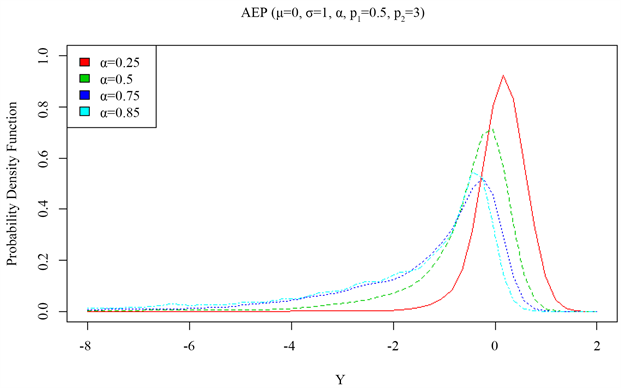Figure 2. Density diagram of asymmetric exponential power distribution (AEPD) with skewness parameter (α)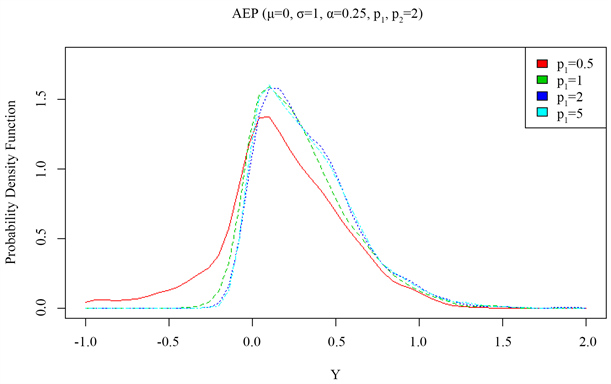Figure 3. Density diagram of asymmetric exponential power distribution (AEPD) as a function of the left tail parameter ( p 1 )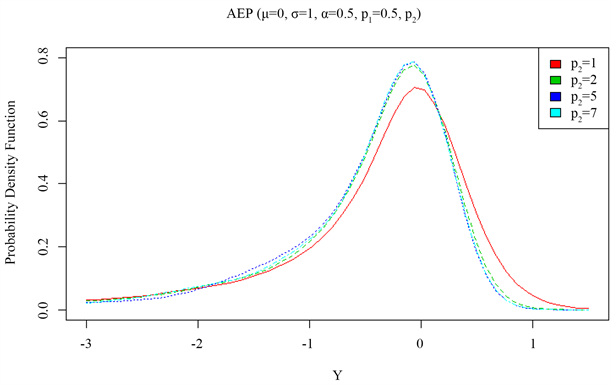Figure 4. Density diagram of asymmetric exponential power distribution (AEPD) with the right tail parameter ( p 2 )Table 1. Comparison table of estimated simulated values and true values following ALD distribution and AEP distribution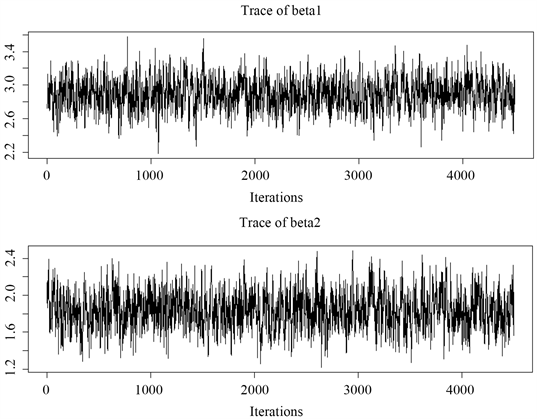Figure 5. Traces of MCMC sampling values of ${\beta }_{1}$ and ${\beta }_{2}$ following ALD distribution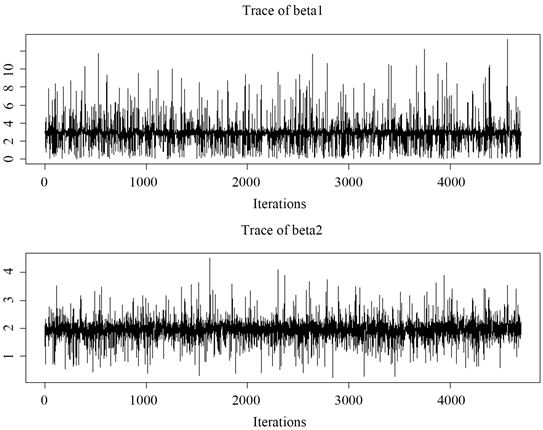Figure 6. Traces of MCMC sampled values of ${\beta }_{1}$ and ${\beta }_{2}$ following AEP distribution

5. 结束语

  Koenker, R. and Bassett Jr, G. (1978) Regression Quantiles. Econometrica, 46, 33-50. https://doi.org/10.2307/1913643  Koenker, R. and Machado, J.A. (1999) Goodness of Fit and Related Inference Processes for Quantile Regression. Journal of the American statistical Association, 94, 1296-1310. https://doi.org/10.1080/01621459.1999.10473882  Yu, K. and Moyeed, R.A. (2001) Bayesian Quantile Regression. Statistics & Probability Letters, 54, 437-447. https://doi.org/10.1016/S0167-7152(01)00124-9  Zhu, D. and Zinde-Walsh, V. (2009) Properties and Estimation of asymmetric Exponential Power Distribution. Journal of Econometrics, 14, 86-99. https://doi.org/10.1016/j.jeconom.2008.09.038  Kozumi, H. and Kobayashi, G. (2011) Gibbs Sampling Methods for Bayesian Quantile Regression. Journal of Statistical Computation and Simulation, 81, 1565-1578. https://doi.org/10.1080/00949655.2010.496117  Naranjo, L., Pérez, C.J. and Martín, J. (2012) Bayesian Analysis of a Skewed Exponential Power Distribution. Proceedings of COMPSTAT, Limassol, 27-31 August 2012, 641-652.  Naranjo, L., Pérez, C.J. and Martín, J. (2015) Bayesian Analysis of Some Models That Use the Asymmetric Exponential Power Distribution. Statistics and Computing, 25, 497-514. https://doi.org/10.1007/s11222-014-9449-1